Latest Banking jobs   »

# Quantitative Aptitude Quiz For SBI Clerk Prelims 2022- 24th May

Q1. Two dices are rolled simultaneously, then find the probability of getting a sum more than 5 or less than 8.
(a) 5/9
(b) 5/12
(c) 3/4
(d) 1/6
(e) 11/36

Quantitative Aptitude Quiz For SBI Clerk Prelims 2022 CHECK IN HINDI

Q2. Ankit Marks a book 25% above Cost price and earn 2.5% Profit. At what discount he should sold the book So that he can earn 5% profit if difference between both selling price was Rs16?
(a) Rs. 132
(b) Rs. 80
(c) Rs. 140
(d) Rs. 128
(e) Rs. 100

Q3. Two trains cross each other in 7 sec while moving in opposite direction and takes 35 sec while moving in same direction. If speed of slower train is 20 m/sec, then find sum of length of both trains?
(a) 350 meters
(b) 400 meters
(c) 250 meters
(d) 200 meters
(e) 550 meters

Q4. Find no. of words can be formed using letters of a English word ‘CIRCUMSTANCES’ so that R and E occupied first and last position respectively.
(a) 13!/3!2!
(b) 13!/6
(c) 11!/12
(d) 11!/3!2!2!
(e) None of these

Q5. Side of an equilateral tringle is equal to diagonal of a square which side is √6 meter. Find Area of equilateral tringle?
(a) 6√3 m²
(b) 12√3 m²
(c) 4√3 m²
(d) 3√6 m²
(e) 3√3 m²

Q6. Ankit and Pankaj invested Rs. 3000 and Rs 5000 for 12 months and 8 months respectively If they donate 71% of their profit and remaining distribute in ratio of their investment. Find profit get by Pankaj out of Rs. 1900?
(a) Rs. 190
(b) Rs. 290
(c) Rs. 280
(d) Rs. 211
(e) Rs. 191

Q7. Find difference between interest earned an Rs. 225 at 20% C.I per years in 2 years and on Rs. 289 at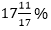at S.I for 1 years?
(a) Rs. 48
(b) Rs. 51
(c) Rs. 99
(d) Rs. 53
(e) Rs. 43

Q8. Tap D can fill 4 buckets in 36 min and tap E can fill 2 buckets in 12 min and tap D and top E can fill a tank in 1 hour. Find Capacity of tank if capacity of a bucket is 12 lit?
(a) 240 lit
(b) 180 lit
(c) 200 lit
(d) 160 lit
(e) None of these

Q9. If 15 books of different name are arranged in an order, find the probability of such order in which book A is kept before book B, book B is kept before book C and book C is kept before book D?
(a) 1/6
(b) 1/24
(c) 1/15
(d) 1/5
(e) Can’t be determine.

Q10. Two train are moving in opposite direction with 15 km/h and S km/h respectively, faster train has length of 150 meter which is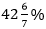of length of slower train. If faster train start chasing slower train 250 meter behind at same time and crosses it in 9 min, then find speed of faster train? (S>15 km/h)
(a) 25 km/h
(b) 16 km/h
(c) 20 km/h
(d) 24 km/h
(e) None of these.

Q11. A shopkeeper marked a book a Rs. 720 and sold at two successive discount 10% and 20%. If cost price of book was Rs. 432, find profit percent?
(a) 22%
(b) 25%
(c) 12%
(d) 37.5%
(e) 20%

Q12. Manoj rows a certain distance from point P to Q and back again to point Q in a river, which flows at rate of 4 km/h, If speed of the man in still water is 12 km/h, then find the Manoj’s average speed for the journey?
(a) 34/3 km/hr
(b) 11 km/hr
(c) 32/3 km/hr
(d) 10 km/hr
(e) None of these

Q13. A and B invested Rs 5000 and Rs. 9000 for 12 months and 6 months respectively. Find profit share of B is how much percent more or less than profit share of A?
(a) 10% less
(b) 11.11% more
(c) 11.11% less
(d) 10% more
(e) 12.5 % more

Q14. A man invested Rs. 3600 for 3 years at 20% per annum on CI, find difference between interest earned in second year and that of in third year?
(a) Rs. 144
(b) Rs. 172
(c) Rs. 172.8
(d) Rs. 152.8
(e) Rs. 180

Q15. Mohit mixes two type of sugar costing Rs 30 per kg and Rs. 35 per kg in ratio of1:4 respectively, if ratio get changed to 4:1, then find how much he will loss for selling mixture of 15 kg at cost price?
(a) Rs. 30
(b) Rs. 45
(c) Rs. 7.5
(d) Rs. 15
(e) None of these.

Solutions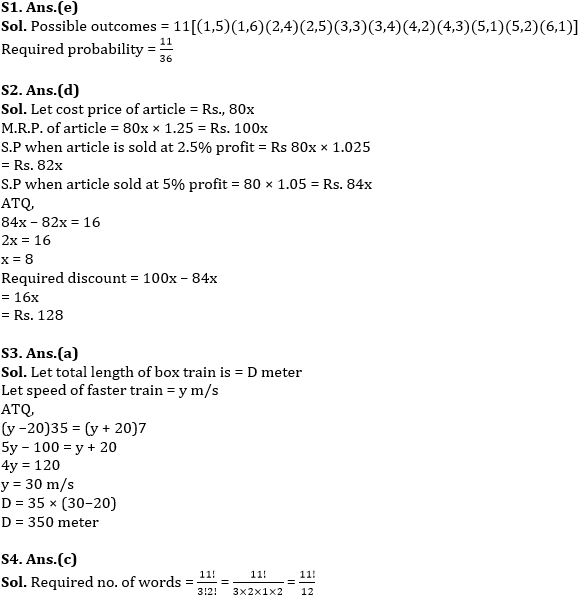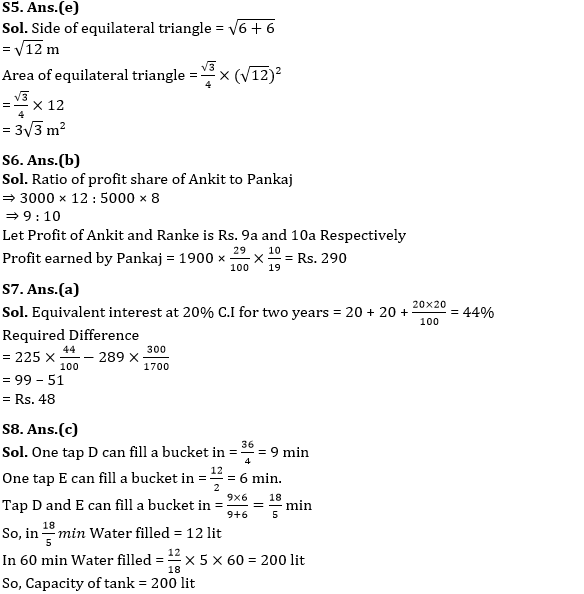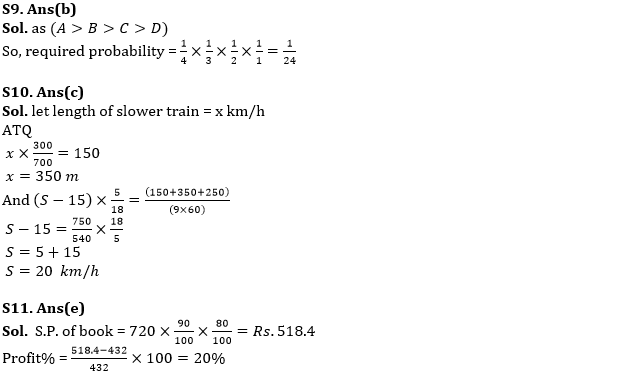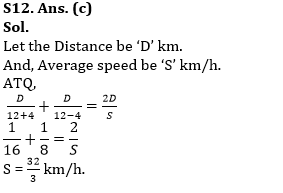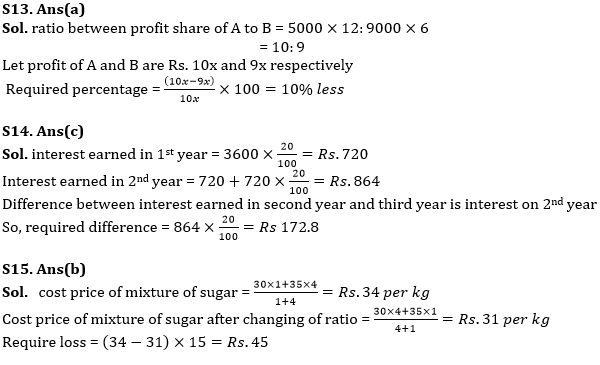#### Congratulations!